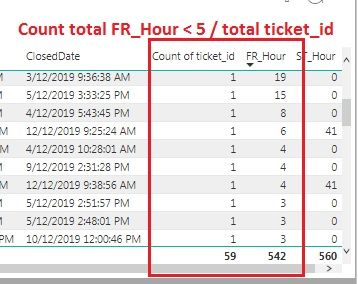cancel
Showing results for
Did you mean:Regular Visitor

## divide with condition less than

Hi All,

Anyone can help me to calculate this formula? I want to get cumulative value (in %) using below dataset.

Ex: (40/59) * 100

40 refer to ticket id which is FR_Hour < 5

59 refer to total ticketThanks

1 ACCEPTED SOLUTIONCommunity Champion

Hi @twister

Try something like this.

``````Measure =
DIVIDE(
CALCULATE(
COUNTROWS( 'Table' ),
'Table'[FR_Hour] < 5 ),
COUNTROWS( 'Table' )
) * 100
``````

Best Regards,
Mariusz

If this post helps, then please consider Accepting it as the solution.

2 REPLIES 2Community Champion

Hi @twister

Try something like this.

``````Measure =
DIVIDE(
CALCULATE(
COUNTROWS( 'Table' ),
'Table'[FR_Hour] < 5 ),
COUNTROWS( 'Table' )
) * 100
``````

Best Regards,
Mariusz

If this post helps, then please consider Accepting it as the solution.Regular Visitor

nice..its work perfectly..thanks